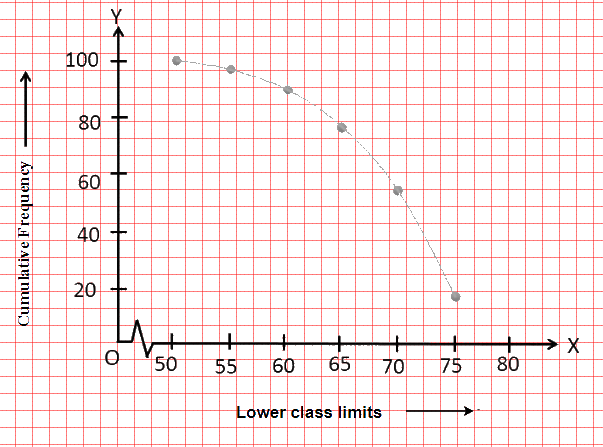# Ex.14.4 Q3 STATISTICS Solution - NCERT Maths Class 10

Go back to  'Ex.14.4'

## Question

The following table gives production yield per hectare of wheat of $$100$$ farms of a village.

 Production yield(in kg/ha) $$50$$ – $$55$$ $$55$$– $$60$$ $$8$$ – $$65$$ $$65$$ – $$70$$ $$70$$ – $$75$$ $$75$$ – $$80$$ Number of farms $$2$$ $$8$$ $$12$$ $$24$$ $$38$$ $$16$$

Change the distribution to a more than type distribution and draw ogive.

## Text Solution

What is known?

Production yield per hectare of wheat of $$100$$ farms of a village.

What is unknown?

The more than type distribution and its ogive.

Reasoning:

The representation of cumulative frequency distribution graphically is known as a cumulative frequency curve or an ogive.

Steps:

The cumulative frequency distribution of more than type can be obtained as follows.

 Production Yield (in kg/ha) (Lower class limits) Cumulative Frequency More than or equal to $$50$$ $$100$$ More than or equal to $$55$$ $$100 - 2 = 98$$ More than or equal to $$60$$ $$98 - 8 = 90$$ More than or equal to $$65$$ $$90 - 12 = 78$$ More than or equal to $$70$$ $$78 - 24 = 54$$ More than or equal to $$75$$ $$54 - 38 = 16$$

Taking the lower-class limits on $$x$$-axis and their respective cumulative frequencies on $$y$$-axis, its ogive can be obtained as follows.Learn from the best math teachers and top your exams

• Live one on one classroom and doubt clearing
• Practice worksheets in and after class for conceptual clarity
• Personalized curriculum to keep up with school